# MATHEMATICS — 2004 (Set I — Compartment Outside Delhi)

SECTION - A

Q.1. Solve forandOr

Solve for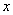and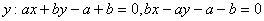Q. 2. If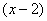(x-2) is a factor of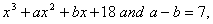findQ. 3. Express the following as a rational expression in simplest form: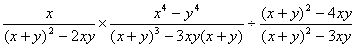Q. 4. Find the sum of first 15 terms of an A.P. whose pith term is 9 - 5 n .

Or

If the sum to first n terms of an A.P. is given by Sn = 5n2+3n, find the n th term of the A.P.

Q. 5. A mixi is available for Rs.1,500 cash or for Rs.360 as cash down payment followed by three monthly instalments of Rs.390 each. Find the rate of interest under the instalment plan.

Q. 6. A loan of Rs.66,200 is to be returned in three equal annual instalments. If the rate of interest is 10% per annum, compounded annually, find each annual instalment.

Q. 7. Prove that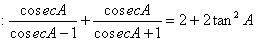Q. 8. Evaluate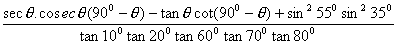Q. 9. Draw a circle of diameter 12 cm. From a point P 10 cm away from its centre, construct a pair of tangents to the circle. Measure the lengths of the tangent segments.

Q. 10. In Fig.1, AB || DE and BD || EF. Prove that DC2 = CF x AC.SECTION B

Q. 11. Solve the following system of linear equations graphicallShade the region bounded by the lines and x-axis.

Q. 12. The monthly incomes of A and B are in the ratio of 9 : 7 and their monthly expenditures are in the ratio of 4 : 3. If each saves Rs.1,600 per month, find the monthly income of each.

Q. 13. The diagonals of a parallelogram ABCD intersect at E. Show that the circumcircles of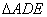and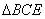touch each other at E.

Q. 14. The angles of depression of the top and bottom of a tower, as seen from the top of a 100 m high cliff, are 30° and 60° respectively. Find the height of the tower.

Q. 15. Pie-chart in Fig. 2, represents the number of votes polled by four candidates in an election. The votes polled by Paramjit Kaur were

160. Read the pie-chart and answer the following questions:

(i) What is the total number of votes polled?
(ii) What is the minimum number of votes polled by a candidate?
(iii) By how many votes did the winner defeat the nearest contestant?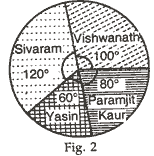Q. 16. Out of 400 bulbs in a box, 15 bulbs are defective. One bulb is taken out at random from the box. Find the probability that the drawn bulb is not defective.

Or

Find the probability of getting 53 Fridays in a leap year.

Q. 17. Find the ratio in which the line-segment joining the points (6, 4) and (1, -7) is divided by x-axis.

Or

The coordinates of two vertices A and B of a triangle ABC are (1, 4) and (5, 3) respectively. If the coordinates of the centroid of AABC are (3, 3). find the coordinates of the third vertex C.

Q. 18. Find the value of m for which the points with coordinates (3, 5), ( m , 6) and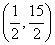are collinear.

Q. 19. 50 circular plates, each of radius 7 cm, and thickness 1/2 cm, are placed one above the other to form a solid right circular cylinder. Find the total surface area and volume of the cylinder so formed. (Use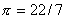)

Q. 20. The following table gives the marks scored by 100 students in a class-test:

 Marks 0-10 10-20 20-30 30-40 40-50 50-60 Number of Student 12 18 27 20 17 6

Find the mean marks scored by a student in the class-test.

SECTION C

Q. 21. A train takes 2 hours less for a journey of 300 km if its speed is increased by 5 km/hour from its usual speed. Find the usual speed of the train.

Or

If twice the area of a smaller square is subtracted from the area of a larger square, the result is 14 cm However, if twice the area of the larger square is added to three times the area of the smaller square, the result is 203 cm 2 Determine the sides of the two squares.

Q. 22. If a line is drawn parallel to one side of a triangle, prove that the other two sides are divided in the same ratio.

Using the above result, prove from Fig. 3, that AD = BE if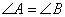and DE || AB.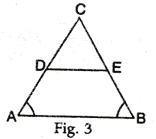Q. 23. Prove that the sum of either pair of opposite angles of a cyclic quadrilateral is 180°. Use the above result to find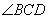in Fig. 4, where O is the centre of the circle and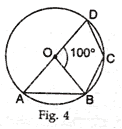Q. 24. A well, of diameter 3 m, is dug 14 m deep. The earth taken out of it has been spread evenly all around it to a width of 4 m, to form an embankment. Find the height of the embankment. (Use)

Or

If the radii of the ends of a bucket, 45 cm high, are 28 cm and 7 cm, find the capacity of the bucket. (Use)

Q. 25. The annual income of Seema (aged 30 years) is Rs.2,20,000 (exclusive of HRA). She contributes Rs.5,000 per month towards provident fund and pays an annual premium of Rs.12,000 towards her LIC policy, She pays Rs.1,000 per month as income tax for the first 11 months. Calculate the income tax payable by her in the last month of the year.

for calculating income tax, use the following:

 (a) Standard Deduction Gross income Deduction (i) Upto Rs.75,000 (ii) From Rs.75,001 to Rs.5,00,000 (iii) More than Rs,5,00,000 40% of gross income Rs.30,000 Rs.20,000 (b) Rates of Taxes Slab Income tax (i) Upto Rs. 50,000 (ii) From Rs. 50,001 to Rs. 60,000 (iii) From Rs. 60,001 to Rs. 1,50,000 (iv) Rs. 1.50,000 and above No tax 10% of the amount exceeding Rs.50,000 Rs.1,000+20% of the amount exceeding Rs.60,000 Rs.19,000+30% of the amount exceeding Rs.1,50,000 (c) Rebate in Tax (i) 20% of the savings subject to a maximum of Rs. 14,000 if the gross income is upto Rs. 1,50,000. (ii)15% of the savings subject to a maximum of Rs. 10,500 if the gross income is from Rs. 1,50,001 to Rs. 5,00,000. (d) Surcharge 10% of the tax payable if theannual income is more than Rs.8,50,000. (e) Rebate for women of less than 65 years Rs. 5,000 over and above other admissible rebates.
Maths 2004 Question Papers Class X
CBSE 2004 Question Papers Class X